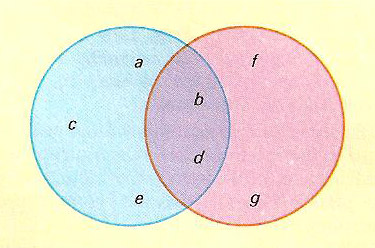A# Venn diagramVenn diagram illustrating two sets, S1 = {a, b, c, d, e} and S2 = {b, d, f, g} for which the intersection of S1 and S2, S1 ∩ S2 = {b, d} and their union, S1 ∪ S2 = {a, b, c, d, e, f, g}.

A Venn diagram is a simple way of representing sets and subsets, which makes use of overlapping circles. Venn diagrams are named after the Englishman John Venn (1834–1923), a fellow of Cambridge University. Venn was a cleric in the Anglican Church, an authority on what was then called "moral science," the compiler of a massive index of all Cambridge alumni, and a rather mundane mathematician who worked in logic and probability theory. The diagrams he used for representing syllogisms appear to have been first called "Venn diagrams" by Clarence Irving in his book A Survey of Symbolic Logic in 1918. However, Venn was lucky to be so immortalized. Both Gottfried Leibniz and Leonhard Euler used very similar forms of representation many years earlier.